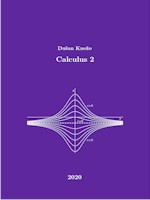# Calculus 2

Just published

 Calculus 2 Dušan Knežo ISBN: 978-3-96595-001-6 Abstract/Contents (free of charge) PDF-file download link 15.00 EUR Printed edition (55.00 EUR) order to RAM-VerlagPreface
This book is a basic source of information for students of mechanical engineering, especially for students of the Faculty of Manufacturing Technologies with a seat in Presov of the Technical University of Kosice. It could also be useful for students of other disciplines if they are interested in the basics of linear algebra, ordinary di erential quations, and the di erential and integral calculus of functions of several variables. The book is divided into four chapters. Chapter 1 is focused on the basics of linear algebra, a substantial part is devoted to matrices and systems of linear equations. Chapter 2 is devoted to the basic types of ordinary di erential equations and their solutions. Chapter 3 is devoted to the di erential calculus of functions of several variables, including applications. Chapter 4 is devoted to double and triple integrals and their geometric and physical applications. Each chapter of this book contains, in addition to the necessary theory, a number of solved examples as well as exercises. It may therefore be a sucient source of information for courses that are similar in content to this book.